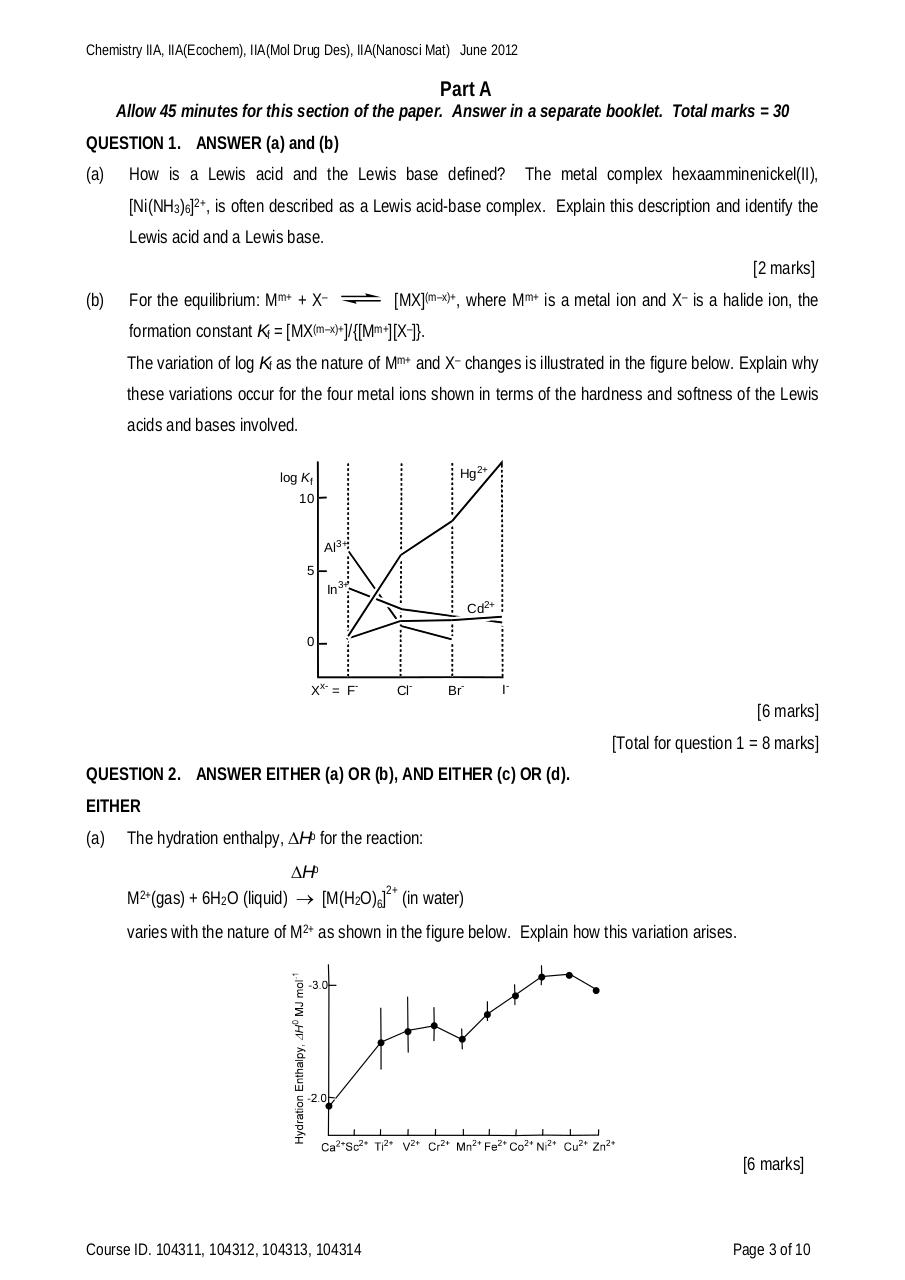# ChemIIA 2012 final.pdfPage 1 2 34517

#### Text preview

Chemistry IIA, IIA(Ecochem), IIA(Mol Drug Des), IIA(Nanosci Mat) June 2012

Part A
Allow 45 minutes for this section of the paper. Answer in a separate booklet. Total marks = 30
QUESTION 1. ANSWER (a) and (b)
(a)

How is a Lewis acid and the Lewis base defined?

The metal complex hexaamminenickel(II),

[Ni(NH3)6]2+, is often described as a Lewis acid-base complex. Explain this description and identify the
Lewis acid and a Lewis base.
[2 marks]
(b)

For the equilibrium: Mm+ + X–

[MX](m–x)+, where Mm+ is a metal ion and X– is a halide ion, the

formation constant Kf = [MX(m–x)+]/{[Mm+][X–]}.
The variation of log Kf as the nature of Mm+ and X– changes is illustrated in the figure below. Explain why
these variations occur for the four metal ions shown in terms of the hardness and softness of the Lewis
acids and bases involved.
Hg2+

log Kf
10

Al3+
5
In3+
Cd2+
0

Xx- = F-

Cl-

Br-

I-

[6 marks]
[Total for question 1 = 8 marks]
QUESTION 2. ANSWER EITHER (a) OR (b), AND EITHER (c) OR (d).
EITHER
(a)

The hydration enthalpy, Ho for the reaction:
Ho
M2+(gas) + 6H2O (liquid)  [M(H2O)6]2+ (in water)
varies with the nature of M2+ as shown in the figure below. Explain how this variation arises.

[6 marks]

Course ID. 104311, 104312, 104313, 104314

Page 3 of 10